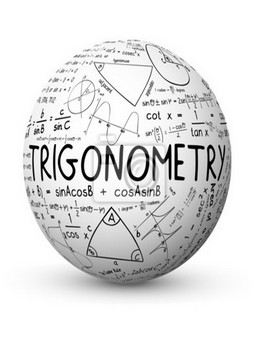# Trigonometry

Trigonometry is considered to be one of the most important and challenging topics of Mathematics segment. Important because it is asked in almost every competitive and government exam and challenging because it contains a wide range of concepts for students to learn. Enroll for the ‘Trigonometry’ Course and learn all the concepts and valuable time saving tricks to solve Questions. Moreover, a wide range of Practice and Test Quizzes are also available in this Course through which you can Excel in this topic.

₹ 20.00
₹ 20.00
Last Update 06/30/2021 25:40 Hours 1
Key Features :
• Course Validity 1 Year
• Short tricks
• Strong -Weak Areas Analysis
• Bi-lingual
• Latest Syllabus 2022-23
• Speed, Accuracy Boosters
• Revision Class
• 200+ Practice Questions

• Trigonometry - Concept, Imp Terms and Functions - 0
• Free preview
• Free preview
• Domain and Range
• Minimum and Maximum Values of Trigonometric Functions
• Important Questions on Trigonometric Ratios
• Practice Questions on Trigonometric Ratios
• Practice Questions on Angle of Elevation
• Practice Questions on Angle of Depression
• Trigonometric Identities - 0
• Trigonometric Identities
• Ratio, Reciprocal, Product, Pythagorean, Angle Identities
• Trigonometric Identities Important Questions
• Trigonometric Identities Practice Session
• Practice Questions on Trigonometric Identities
• Trigonometry - Important Formulas - 0
• Complementary Angles and Quadrant Formula - Session 1
• Quadrant Formula - Session 2
• Sum and Difference Formula
• Product/Sum/Difference/Double Half/Thrice Angle Formulas
• Question Session on Product/Sum/Difference/Double Half/Thrice Angle Formulas
• Trigonometry - Important Laws and Rules - 0
• Sine and Cosine Laws in Triangle - Session 1
• Sine and Cosine Laws in Triangle - Session 2
• Projection Rule, Napier's Analogy and Area of Triangle
• Radian and Degree Based Questions
• Practice Questions on Circular Measure of Angle
• Trigonometry - Mixed Important Questions - 0
• Mixed Question Session 1
• Mixed Question Session 2
• Mixed Question Session 3
• Important Questions on Identities - Class 1
• Important Questions on Identities - Class 2
• Trigonometry - Practice Quizzes - 0
• Practice Quiz 1 - Easy level Questions
• Practice Quiz 2 - Moderate level Questions
• Practice Quiz 3 - Advanced level Questions
• Trigonometry - Concept, Imp Terms and Functions
• Trigonometric Identities
• Trigonometry - Important Formulas
• Trigonometry - Important Laws and Rules
• Trigonometry - Mixed Important Questions
• Trigonometry - Practice Quizzes